# java多线程同步 synchronized

86 篇文章 2 订阅

## 多线程数数

1. 第一种实现
package org.fan.learn.synchronize;
}
@Override
public synchronized void run() {
for (int i = 0; i < 10; i++) {
System.out.println("No." + threadNo + " : " + i);
}
}

public static void main(String[] args) {
for (int i = 0; i < 5; i++) {
}
}
}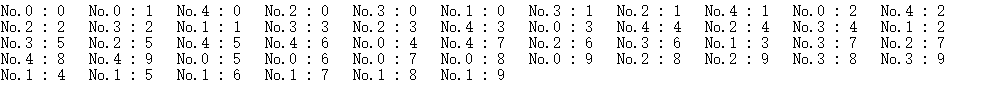2.第二种实现

package org.fan.learn.synchronize;

private String lock;
this.lock = lock;
}

@Override
public void run() {
synchronized (lock) {
for (int i = 0; i < 10; i++) {
System.out.println("No." + threadNo + " : " + i);
}
}
}

public static void main(String[] args) {
String lock = new String("lock");
for (int i = 0; i < 5; i++) {
}
}
}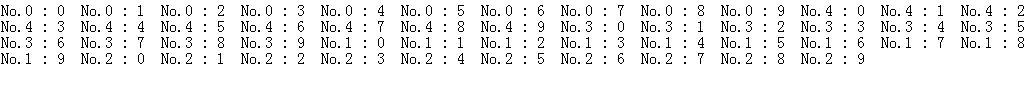3.第三种实现

package org.fan.learn.synchronize;
}
private static synchronized void abc(int threadNo) {
for (int i = 0; i < 10; i++) {
System.out.println("No." + threadNo + " : " + i);
}
}
@Override
public void run() {
}

public static void main(String[] args) {
for (int i = 0; i < 5; i++) {
}
}
}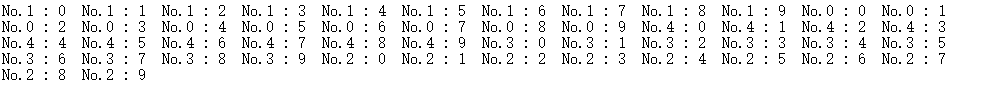## 多线程累加

1. 第一种实现
代码如下：
package org.fan.learn.synchronize;
class Count {
private int num;
public Count() {
this.num = 0;
}
public void count() {
for (int i = 0; i < 10; i++) {
num += i;
}
}
}

public static void main(String[] args) {
Runnable runnable = new Runnable() {
public void run() {
count.count();
}
};
for (int i = 0; i < 5; i++) {
}
}
}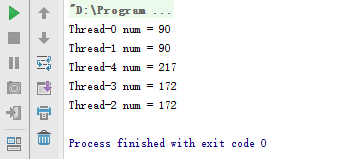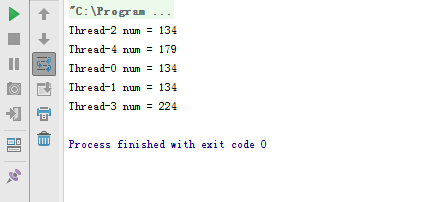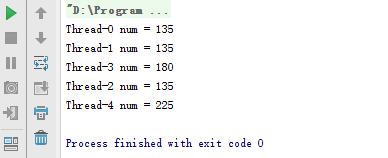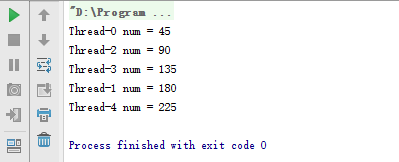2.第二种实现

package org.fan.learn.synchronize;

class Count {
private int num;
public Count() {
this.num = 0;
}
public synchronized void count() {
for (int i = 0; i < 10; i++) {
num += i;
}
}
}

public static void main(String[] args) {
Runnable runnable = new Runnable() {
public void run() {
count.count();
}
};
for (int i = 0; i < 5; i++) {
}
}
}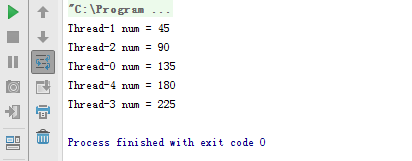3.第三种实现

package org.fan.learn.synchronize;

class Count {
private int num;
public Count() {
this.num = 0;
}
public void count() {
for (int i = 0; i < 10; i++) {
num += i;
}
}
}

public static void main(String[] args) {
Runnable runnable = new Runnable() {
public void run() {
count.count();
}
};
for (int i = 0; i < 5; i++) {
}
}
}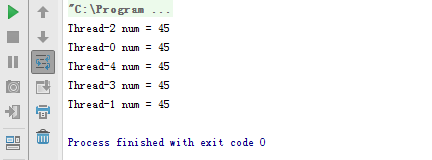4.第四种实现

package org.fan.learn.synchronize;

class Count {
public void count() {
int num = 0;
for (int i = 0; i < 10; i++) {
num += i;
}
}
}

public static void main(String[] args) {
Runnable runnable = new Runnable() {
public void run() {
count.count();
}
};
for (int i = 0; i < 5; i++) {
}
}
}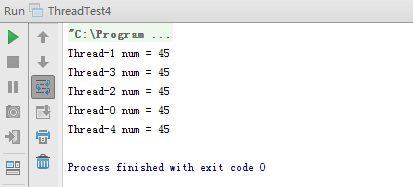5.第五种实现

package threadlocal;

/**
* Created by fan on 15-12-2.
*/
public class Count {
@Override
protected Integer initialValue() {
return 0;
}
};

public Integer count() {
for (int i = 0; i < 10; i++) {
num.set(num.get() + i);
}
return num.get();
}
}
package threadlocal;

/**
* Created by fan on 15-12-2.
*/

private Count count;

this.count = count;
}

public void run() {
}

public static void main(String[] args) {
Count count = new Count();
for (int i = 0; i < 5; i++) {
}
}
}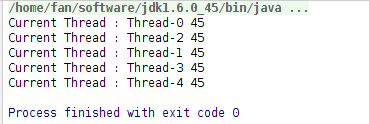06-299749
11-23268812-0693
03-295325
11-068036
03-291万+
03-301万+
04-073万+
04-081万+
06-195047
12-02397
05-044603
01-117334
04-071万+
11-196423
04-089061
04-244819
04-085143
12-06142
12-04135点击重新获取扫码支付余额充值